Mach–Zehnder interferometer with squeezed and EPR entangled optical fields
Yu Xu-Dong1, 3, Li Wei1, 3, Zhu Shi-Yao2, Zhang Jing1, †,The State Key Laboratory of Quantum Optics and Quantum Optics Devices, Institute of Opto-Electronics, Shanxi University, Taiyuan 030006, China
Beijing Computational Science Research Center, Beijing 100084, China
Collaborative Innovation Center of Extreme Optics, Shanxi University, Taiyuan 030006, China

† Corresponding author. E-mail: E-mail:jzhang74@yahoo.com, jzhang74@sxu.edu.cn

Project supported by the National Basic Research Program of China (Grant No. 2011CB921601), the National Natural Science Foundation of China (Grant Nos. 11234008, 11361161002, and 61571276), and the Natural Science Foundation of Shanxi Province, China (Grant No. 2015011007).

Abstract
Abstract

We study a scheme for Mach–Zehnder (MZ) interferometer as a quantum linear device by injecting two-mode squeezed input states into two ports of interferometer. Two-mode squeezed states can be changed into two types of inputs for MZ interferometer: two squeezed states and Einstein–Podolsky–Rosen (EPR) entangled states. The interference patterns of the MZ interferometer vary periodically as the relative phase of the two arms of the interferometer is scanned, and are measured by the balanced homodyne detection system. Our experiments show that there are different interference patterns and periodicity of the output quantum states for two cases which depend on the relative phase of input optical fields. Since MZ interferometer can be used to realize some quantum operations, this work will have the important applications in quantum information and metrology.

1. Introduction

The interferometers are among the most sensitive devices for metrology and weak forces detection. Most interferometers can be mapped on the Mach–Zehnder (MZ) configuration.[1,2] An input field is split by the first beamsplitter (BS) of the interferometer into two, which enter into two arms where their phase difference is generated by passing though an object in one of the two arms, and then are recombined with second BS and the resultant interference pattern will be observed which contains the information of the object. If a coherent field is fed into the primary input port of the first BS and the vacuum is injected into the second port, the sensitivity of the interferometer is limited to the shot noise limit (SNL)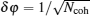, where Ncoh is the average number of photons in the coherent beam. This limit may be surpassed towards the Heisenberg limit δφ = 1/N, if quantum states are used as the input. When the squeezed vacuum is injected into unused port, it can increase a phase sensitivity below the shot noise limit. Such a method has been applied to laser interferometers for gravitational wave detection.[6,7] Furthermore MZ interferometer can be used as a quantum linear device to realize the quantum transformation by injecting quantum states into two ports of interferometer, for example, using the maximally entangled NOON state, which can improve the sensitivity in the phase measurement.

In this paper, we experimentally study MZ interferometer as a quantum linear device with two-mode squeezed input states. Two-mode squeezed states can be transformed into two types of inputs: (i) the squeezed states and (ii) the Einstein–Podolsky–Rosen (EPR) entangled states. The interference of two outputs from a BS for the two squeezed states and the EPR entangled states has been studied and used in CV quantum information and communication, such as quantum teleportation,[13,14] quantum dense coding.[15,16] The MZ interferometer with two-mode squeezed states has also been theoretically investigated. However, the related experiment has not been reported, which is important in quantum information and metrology.

2. Theoretical analysis

Although the two squeezed states and the EPR entangled states can be transformed each other easily by a beam splitter, they present the quite different interference patterns and periodicity when they are injected into the MZ interferometer. In this paper, the two squeezed states (or EPR entangled states) with an adjusted relative phase are injected into the two ports of the MZ interferometer. The interference patterns of the MZ interferometer vary periodically as the phase difference of the two arms of the interferometer is scanned, and are measured by the balanced homodyne detection system. In the case of the EPR entangled states, the output states of the MZ interferometer are changed from squeezed states into entangled states with the periodicity of λ/2. The interference pattern will be only shifted keeping the same shape when the input relative phase is changed. In the case of the two squeezed states, the interference pattern of the output states depend on the input relative phase of two squeezed states. We also employ a coherent excited squeezed state in phase space as one of two input squeezed states and study the interference pattern of the MZ interferometer.

We first consider the simple case, in which two quantum optical beams interfere at a 50/50 BS. The input fields of BS can be written as i = (ai + iai)/2, i = 1,2 denote the two input ports and ai and ai are the corresponding quadrature amplitude and quadrature phase operators with the canonical commutation relation [ai,ai] = 2i. The two output fields of the BS are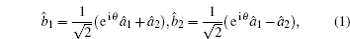where θ is the phase difference of the two beams. When the relative phase θ is varied, the output states will be changed, as shown in Fig. 1. The arbitrary quadrature component of the output states may be measured by homodyne detection. The periodicity of 2π corresponds to the wave length λ of the input light fields. We will discuss: i) the squeezed states and ii) the EPR entangled input states, respectively.

 Figure Option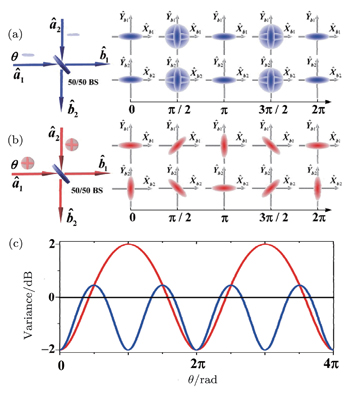Fig. 1. Schematic diagram of the quantum interference of two-mode squeezed states on a 50% BS. (a) left: schematic diagram of the interference of the squeezed state light fields coupled into the 50% beam splitter; right: the error contours of the output states versus the phase difference θ in phase space. (b) left: schematic diagram of the entangled state light fields entering the 50% beam splitter; right: the error contours of the output states versus θ in phase space. (c) Theoretical calculation of the variance of quadrature phase of the output state. Blue line, red line and black line correspond to the two squeezed states, entangled states and SNL, respectively.

For the two squeezed-states light fields as the input states of a 50/50 BS, the variances of the input fields can be expressed as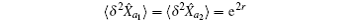and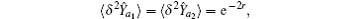where r is the squeeze parameter. When θ = (n = 0,1,2,…), the variances of the quadrature components of the output fields are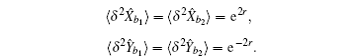Obviously, the output fields are squeezed states. When θ = + π/2, we can obtain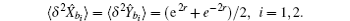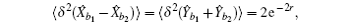which shows the two output states are EPR entangled states.[20,21] Thus, when the relative phase θ is scanned, the output states from a 50/50 BS are changed from squeezed states into entangled states with the periodicity of π as shown in Fig. 1(a).

Considering the interference of the entangled states on a 50/50 BS. The quadratures of the states in the two arms after the interference are obtained according to Eq. (1)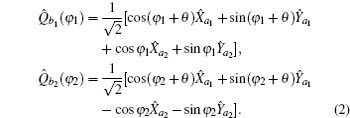Here, a(φ) = e−iφ + eiφ and φ the angle of quadrature component, so a(0) = a and a(π/2) = a. From Eq. (2), we can see that the outputs of two arms after the 50/50 BS always keep the squeezed states. The squeezing angles of two arms are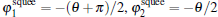in phase space respectively, and the squeezed quadrature variance are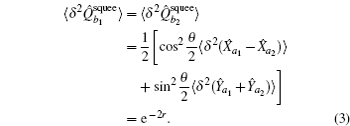It is clearly shown that the angles of the squeezing states rotate with respect to the input relative phase θ, as shown in Fig. 1(b). Moreover the angles of the squeezing states for two arms are orthogonal. The quadrature variance of one arm of the BS with the homodyne detection is shown in Fig. 1(c) with red solid line.

Now we consider the MZ interferometer with two types of input quantum optical fields as shown in Fig. 2. The relative phases θ is added before the input quantum states entering the MZ interferometer. The relative phase ϕ is introduced in one of the two arms of the interferometer. Please note the two phases, θ and ϕ are independent. The two beams in the two arms are recombined and interfere on BS2 of the MZ interferometer. For the input of the EPR entangled states, the quantum states after first BS of the MZ interferometer are always squeezed states, which have the different squeezing angles for the different input relative phases θ. Thus, the output states of the MZ interferometer are changed from squeezed states into entangled states with the periodicity of λ/2 when the relative phase ϕ of the two arms is scanned. The interference pattern of the MZ interferometer is only shifted when the input relative phase, ϕ is changed. For the input of the two squeezed states, the quantum states after first BS of the MZ interferometer are changed from squeezed states into entangled states for the different input relative phases θ. Thus the interference pattern of the MZ interferometer depends on the input relative phase θ of the two squeezed states.

 Figure Option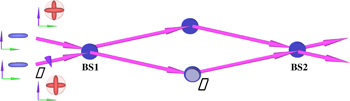Fig. 2. Schematic diagram of the MZ interferometer with two types of input quantum optical fields. The two input optical fields are interfere in the first BS with the different input relative phases θ. Then they are recombined and interfere on the second BS with the relative phase ϕ of the two arms of the interferometer.
3. Experiment and results

The experimental setup is shown in Fig. 3. Two degenerate optical parametric amplifiers (OPA’s) are pumped by a green laser 532 nm and are feeded by the infrared laser 1064 nm.[22,23] The light is a diode-pumped intracavity frequency-doubled (continuous-wave ring Nd:YVO4 + KTP) laser. The periodically poled KTP (PPKTP) crystals are used in the OPA’s, whose temperatures are controlled by the temperature controllers. The OPA’s are concentric resonators. The lengths are about 60 mm. The input and output mirrors are concave, whose curvature radius are 30 mm. The input couplers of the two OPA’s have the same reflections of about 99.5% at 1064 nm, and have the transmissions of 70% and 80% at 532 nm, respectively. The transmissions of the output mirrors of the OPA1 and OPA2 are 13% and 11% at 1064 nm and the reflections are about 99.5% at 532 nm. Two OPA’s are all operated below threshold with the pump fields of 89 mW and 102 mW. Two squeezed lights can be obtained simultaneously with more than −1.5 dB squeezing and 7 dB anti-squeezing. The two squeezed lights from two OPA’s interfere in an equivalent 50% BS, which consists of two polarized beam splitters (PBS) and a half wave plate (HW). The input relative phase θ of two squeezed states can be adjusted by PZT1. Two squeezed lights with linearly horizontal and perpendicular polarization combine in the PBS1 and interfere in PBS2 when setting the angle of HW1 at 22.5°. Then two beams pass through two arms with the almost equal length of ∼ 19 cm and interfere on the second 50% BS2 with the same polarization. The optical path difference of the two arms are scanned by M3 mounted on PZT2. This configuration forms the MZ interferometer with two input squeezed states. For the case of the input EPR entangled states, the EPR entangled state first is generated by interfering two squeezed state in an equivalent 50% BS. The reflective mirror M4 has a small transmission of about 2%, and we can lock the relative phase of the two squeezed-state lights at π/2 by feeding the amplified electronic signal of the interference fringe from detector (D1) back to the PZT1. Then EPR entangled state pass through the MZ interferometer, which consists of BS2 and another 50% BS (which is not shown in Fig. 3).

 Figure Option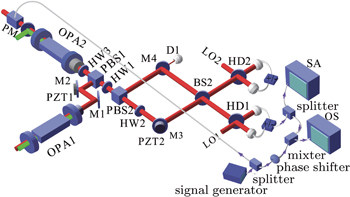Fig. 3. Schematic diagram of the experimental setup. The MZ interferometer with two input squeezed states consists of two PBSs, i.e., PBS1 and PBS2. The MZ interferometer with EPR entangled state consists of BS2 and another BS. OPA: optical parametric amplifiers; M1–M3: high-reflective mirrors; PZT: piezoelectric transducers; PBS: polarized beam splitters; HW: half wave plates; BS: 50% beam splitter; HD: balanced homodyne detectors; LO: Local oscillator; SA: Spectrum analyzer; OS: Oscilloscope; PM: phase modulator.

The two output fields can be measured respectively by the balanced homodyne detection system. Here we also record the noise fluctuation directly, this technology is used in quantum tomography. We divide the electronic signal from the balanced homodyne detection system 1 (HD1) into two parts. One of them is used to measure the quadrature variance by the spectrum analyzer at the analyzed frequency of 6 MHz. The modulation frequency is much smaller than the line-width (∼70 MHz) of the cavity. The other is mixed with a sinusoidal signal at 6 MHz from a function generator, the output signal of the mixer is passed through a low pass filter (Mini-circuit Model BLP 1.9 MHz). At last the low-frequency electric signal is recorded by a high-speed digital oscilloscope (6 × 105 points for per trace), when the relative phase of the two arms of the interferometer is swept by 2π (corresponding to λ) in 120 ms.

For the input of the two squeezed states, the interference patterns of the MZ interferometer are measured with the input relative phases θ as 0°, 30°, 60°, 90°, as shown in Fig. 4. Here, the relative phase ϕ of the two arms of the interferometer is scanned, and the quadrature component of a output field is measured by fixing the relative phase π/2 of the detected light and the local oscillator in the homodyne detection system. For the input relative phases θ = 0°, the quantum states after first BS of the MZ interferometer are still two squeezed light. Thus the two squeezed light will interfere in the second BS of the MZ interferometer, and the output states are changed from squeezed states into entangled states with the periodicity of π, which correspond to the interference patterns of the MZ interferometer as shown in Fig. 4(a). The variance corresponding to Fig. 4(a) is given in Fig. 4(f) (blue line). For θ = 30° and 60°, the quantum states after first BS of the MZ interferometer fall in between the squeezed and EPR entangled states. The interference patterns of the MZ interferometer as shown in Figs. 4(b) and 4(c) respectively, whose variances are given in Fig. 4(f) (green and pink line respectively). For θ = 90°, the quantum state after first BS of the MZ interferometer become EPR entangled light. The periodicity of the interference patterns of the MZ interferometer is 2π as shown in Fig. 4(d), whose variances are given in Fig. 4(f) (red line). It is clearly shown that the output states of the MZ interferometer maintain two squeezed states, however, the angles of the squeezing states rotate with respect to the relative phase ϕ of the two arms.

 Figure Option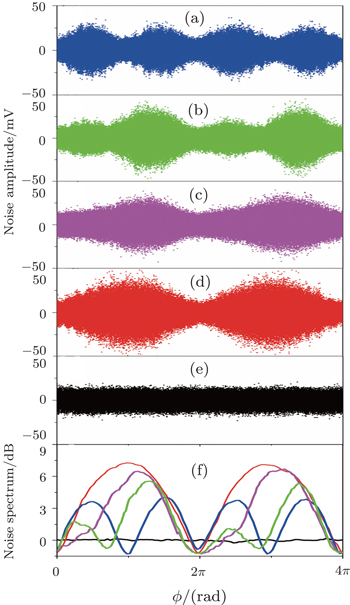Fig. 4. Experimental results of the interference patterns of the MZ interferometer. The interference patterns (noise traces) of the MZ interferometer for two input squeezed states are given in (a) with the input relative phases θ = 0°, (b) 30°, (c) 60°, (d) 90°. Panel (e) is noise trace for two input vacuum state. Panel (f) is the variances of quantum states. Blue line corresponds to panel (a), green line to panel (b), pink line to panel (c), red line to panel (d), and dark line to panel (e).

For the input of the EPR entangled state, the quantum states after first BS of the MZ interferometer are always squeezed states, which have the different squeezing angles for the different input relative phases θ. Thus, the interference patterns of the MZ interferometer is the same as that of two input squeezed states with the input relative phases θ = 0° (Fig. 4(a)). The interference patterns of the MZ interferometer is only shifted when the input relative phase is changed.

Here, we employ a coherent excited squeezed state in phase space as one of two input squeezed states of the MZ interferometer. A subharmonic laser 1064 nm from the main laser beam is phase-modulated by an electro-optic modulator at the frequency 6 MHz (This signal is from the same sinusoidal wave at 6 MHz in homodyne detection), and injected into the OPA2 through its high reflector. As the relative phase between seed wave and pump wave is controlled by a mirror attached to a piezoelectric actuator, the coherent excited squeezed state is generated (OPA operating at amplification). The output of OPA1 is still squeezed vacuum state. Two squeezed states are injected into the MZ interferometer and the interference patterns are shown in Fig. 5.

 Figure Option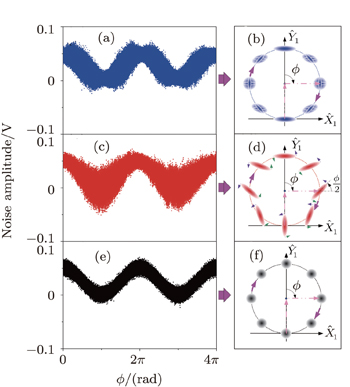Fig. 5. Experimental results of the interference patterns of the MZ interferometer with coherent excited two-mode squeezed states. Panel (a) is the input relative phases θ = 0° and panel (b) is the evolutionary of the output quantum states in phase space as the function of the relative phase ϕ of two arms of the interferometer, corresponding to panel (a). Panel (c) is for 90° and panel (d) is the quantum state evolution in phase space corresponding to panel (c). Panel (e) is noise trace of the MZ interferometer with an input coherent state and an input vacuum state and panel (f) is the state evolution in phase space corresponding to panel (e).

The average photon number of the input optical fields of MZ interferometer can be obtained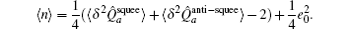Here,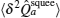and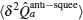are squeezing and anti-squeezing of the optical field, respectively. e0 is the coherent excited amplitude at two sidebands ω0 ± 6 MHz, which can be determined from Fig. 5 and is scaled according to the shot noise limit (the unit is the standard deviation of the shot noise limit). The experimental parameters of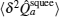andare 0.71 and 5 respectively (corresponding to squeezing -1.5 db and anti-squeezing 7 dB). The coherent excited amplitude is e0 = 3.2. Hence The average photon numbers 〈n〉 of the coherent excited squeezed state and squeezed vacuum are 3.5 and 0.93 respectively.

Figure 5(a) shows the noise trace with the input relative phases θ = 0°, which indicate that the noise trace of the MZ interferometer of two input squeezed vacuum states superimposes on a sinusoidal curve with the periodicity of 2π. This sinusoidal curve comes from the coherent excitation. Here the evolution of the output quantum states in phase space as the function of the relative phase ϕ of the two arms of the interferometer is also plotted in Fig. 5(b). Figure 5(c) shows the noise trace with the input relative phases θ = 90°, and the evolution of the output quantum states in phase space is given in Fig. 5(d). Figures 5(e) and 5(f) show that the one is input coherent state and the other is input vacuum state.

4. Conclusion

In conclusion, we experimentally study the quantum interference on an MZ interferometer with the two-mode squeezed states. The output quantum states are measured by the balanced homodyne detection system and the detailed information of quantum states are given by the noise trace and variance. There are different interference patterns and periodicity for two cases which depend on the relative phase of input optical fields. We believe this work can be used in the quantum information and measurement. For example, quantum interference on MZ interferometer with an unbalanced arm length[26,27] for the broadband squeezed and EPR entangled fields, which is sensitive to the different frequencies of sideband modes of the broadband fields and generate the frequency-dependence squeezing or entanglement, will be an interesting topic in the future.

Reference
 1 Zehnder L1891Z. Instrumentenkd11275 2 Mach L1892Z. Instrumentenkd1289 3 Caves C M 1981 Phys. Rev. D 23 1693 4 Xiao MWu L AKimble H J 1987 Phys. Rev. Lett. 59 278 5 Lang M DCaves C M 2013 Phys. Rev. Lett. 111 173601 6 Vahlbruch HChelkowski SHage BFranzen ADanzmann KSchnabel R 2005 Phys. Rev. Lett. 95 211102 7 The LIGO Scientific Collaboration2011Nat. Phys.796210.1038/nphys2083 8 Boto A NKok PAbrams D SBraunstein S LWilliams C PDowling J P 2000 Phys. Rev. Lett. 85 2733 9 Kok PLee HDowling J P 2002 Phys. Rev. A 65 052104 10 Walther PPan J WAspelmeyer MUrsin RGasparoni SZeilinger A 2004 Nature 429 158 11 Mitchell M WLundeen J SSteinberg A M 2004 Nature 429 161 12 Nagata TOkamoto RO’Brian J LSasaki KTakeuchi S 2007 Science 316 726 13 Furusawa ASørensen J LBraunstein S LFuchs C AKimble H JPolzik E S 1998 Science 282 706 14 Bowen W PTreps NBuchler B Cet al. 2003 Phys. Rev. A 67 032302 15 Li XPan QJing JZhang JXie CPeng K 2002 Phys. Rev. Lett. 88 047904 16 Mizuno JWakui KFurusawa ASasaki M 2005 Phys. Rev. A 71 012304 17 D’Ariano G MPresti P LParis M G A 2001 Phys. Rev. Lett. 87 270404 18 Anisimov P MRateman G MChiruvelli Aet al. 2010 Phys. Rev. Lett. 104 103602 19 Zhang YLi XJin G 2013 Chin. Phys. B 22 114206 20 Simon R 2000 Phys. Rev. Lett. 84 2726 21 Duan L MGiedke GCirac J IZoller P 2000 Phys. Rev. Lett. 84 2722 22 Di KYu XZhang J2010Acta Sin. Quantum Opt.16241 23 Yu XLi WJin YZhang J 2014 Sci. China-Phys. Mech. Astron. 57 875 24 Breitenbach GSchiller SMlynek J 1997 Nature 387 471 25 Glöckl OAndersen U LLorenz SSilberhorn CKorolkova NLeuchs G 2004 Opt. Lett. 29 1936 26 Su XTan AJia XPan QXie CPeng K 2006 Opt. Lett. 31 1133 27 Zhang J 2003 Phys. Rev. A 67 54302 28 Chelkowski SVahlbruch HHage BFranzen ALarsten NDanzmann KSchnabel R 2005 Phys. Rev. A 71 013806